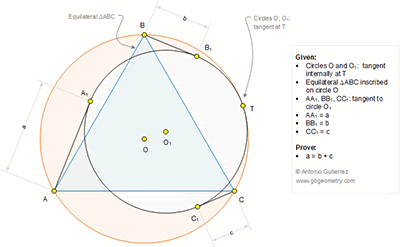## Sunday, October 23, 2016

### Geometry Problem 1276 Equilateral Triangle, Circumcircle, Internally Tangent Circles, Tangent Line, Measurement

Geometry Problem. Post your solution in the comment box below.
Level: Mathematics Education, High School, Honors Geometry, College.

Click the figure below to view more details of problem 1276.#### 4 comments:

1.ABTC is cyclic quadrilateral, By Ptolemy's theorem AT*BC = BT*AC + CT*AB,
Since AB=BC=AC, we have AT = BT + CT,
Lets assume AT,BT and CT meets inner circle O1 at X,Y and Z. We can see that Angle XTY = Angle XTZ = 60 Deg, also Angle YTZ=120 Deg, hence Triangle XYZ is equilateral.

Also Angle ATO = Angle XTO1, hence angle subtended by AT and XT on circle O and O1 are equal. We have XT/AT=YT/BT=ZT/CT=r/R (r is radius of inner circle O1, and R is radius of outer circle O).
Subtracting each term from 1. We get AX/AT=BY/BT=CZ/CT=1-(r/R)=k
AA1^2=AX.AT=k.AT^2 or AA1=sqrt(k).AT,similarly BB1=sqrt(k).BT,CC1=sqrt(k).CT
Since AT=BT+CT we get sqrt(k).AT=sqrt(k).BT + sqrt(k).CT or AA1=BB1+CC1

2.Problem 1276
We apply the Casey’s theorem for zero circles A, B, C, and the circle with center O_1.Then
AC.AA_1=AC.BB_1+AB.CC_1 or AA1=BB1+CC1.(AB=BC=AC).
APOSTOLIS MANOLOUDIS 4 HIGH SCHOOL KORYDALLOS PIRAEUS GREECE

1.Great!!!
Very nice solution.

-Pradyumna

2.Pradyumna thank you very much and your own solution is very good.